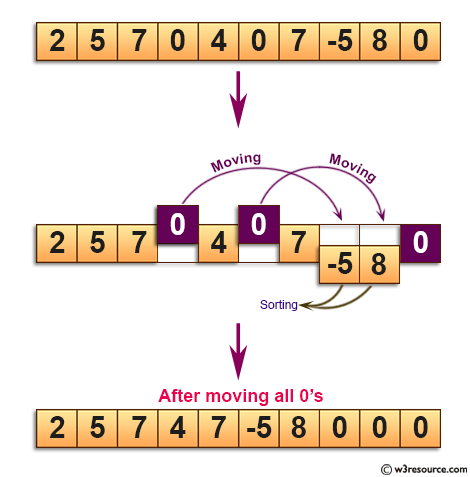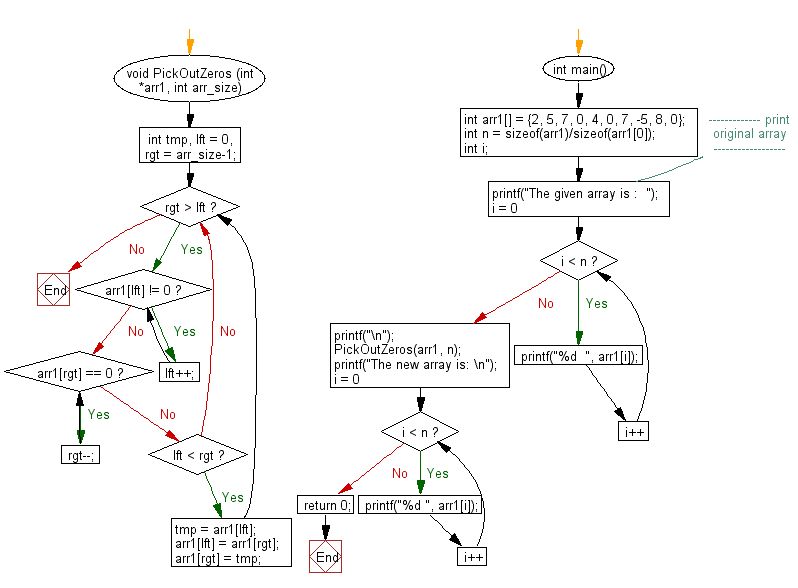﻿ C exercises: Move all zeroes to the end of a given array - w3resource# C Exercises: Move all zeroes to the end of a given array

## C Array: Exercise-58 with Solution

Write a program in C to move all zeroes to the end of a given array.

Pictorial Presentation:Sample Solution:

C Code:

``````#include <stdio.h>

void PickOutZeros (int *arr1, int arr_size)
{
int tmp, lft = 0, rgt = arr_size-1;
while(rgt > lft)
{
while(arr1[lft] != 0)
lft++;
while(arr1[rgt] == 0)
rgt--;
if(lft < rgt)
{
tmp = arr1[lft];
arr1[lft] = arr1[rgt];
arr1[rgt] = tmp;
}
}
}

int main()
{
int arr1[] = {2, 5, 7, 0, 4, 0, 7, -5, 8, 0};
int n = sizeof(arr1)/sizeof(arr1);
int i;
//------------- print original array ------------------
printf("The given array is :  ");
for(i = 0; i < n; i++)
{
printf("%d  ", arr1[i]);
}
printf("\n");
//------------------------------------------------------
PickOutZeros(arr1, n);
printf("The new array is: \n");
for(i = 0; i < n; i++)
{
printf("%d ", arr1[i]);
}
return 0;
}
```
```

Sample Output:

```The given array is :  2  5  7  0  4  0  7  -5  8  0
The new array is:
2 5 7 8 4 -5 7 0 0 0
```

Flowchart:C Programming Code Editor:

Improve this sample solution and post your code through Disqus.

What is the difficulty level of this exercise?

﻿